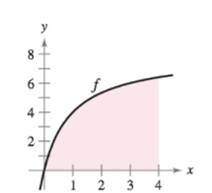Chapter 4.2, Problem 71E

Chapter
Section
Textbook Problem

Graphical Reasoning Consider the region bounded by the graphs of f ( x ) = 8 x / ( x + 1 ) , x = 0 , x = 4 , and y = 0 , as shown in the figure. To print an enlarged copy of the graph, go to MathGraphs.com.(a) Redraw the figure, and complete and shade the rectangles representing the lower sum when n = 4 . Find this lower sum.(b) Redraw the figure, and complete and shade the rectangles representing the upper sum when n = 4 . Find this upper sum.(c) Redraw the figure, and complete and shade the rectangles whose heights are determined by the function values at the midpoint of each subinterval when n = 4 . Find this sum using the Midpoint Rule.(d) Verify the following formulas for approximating the area of the region using n subintervals of equal width.Lower sum: s ( n ) = ∑ i = 1 n f [ ( i − 1 ) 4 n ] ( 4 n ) Upper sum: S ( n ) = ∑ i = 1 n f [ ( i ) 4 n ] ( 4 n ) Midpoint Rule: M ( n ) = ∑ i = 1 n f [ ( i − 1 2 ) 4 n ] ( 4 n ) (e) Use a graphing utility to create a table of values of s(n),S(n), and M(n) for n = 4 , 8 , 20 , 100 , and 200.(f) Explain why s(n) increases and S(n) decreases for increasing values of n, as shown in the table in part (e).

(a)

To determine

To Graph: The region bounded by the graphs of y=8x(x+1),y=0,x=0, and x=4 and shade the rectangles representing the lower sum when n=4. Also, find the lower sum.

Explanation

Given:

Graph:

From the graph the interval of the region is [0,4].

Now, partition of interval [0,4] into 4 subintervals each of width:

Δx=ban=404=1

Because, the given function is increasing, so, the minimum value on each subinterval occurs at the left endpoints.

Therefore, the rectangles representing the lower sum when n=4 is:

It forms only three rectangles instead of four because first left point will start from zero. Therefore, no rectangle is formed at 0.

The lower sum is:

s(n)=i=1nf(xi1)Δx

For number of divisions equal to 4, the lower sum is calculated by

s(n)=i=14f(xi1</

(b)

To determine

To Graph: The region bounded by the graphs of y=8x(x+1),y=0,x=0, and x=4 and shade the rectangles representing the upper sum when n=4. Also, find the upper sum.

(c)

To determine

To Graph: The region bounded by the graphs of y=8x(x+1),y=0,x=0, and x=4 and shade the rectangles whose heights are determined by the function values at the midpoint of each subintervalwhen n=4. Also, find the sum by midpoint rule.

(d)

To determine

To Prove: The formula for:

Upper sum S(n)=i=1n[(i)(4n)](4n)

Lower sum s(n)=i=1n[(i1)(4n)](4n)

Midpoint rule M(n)=i=1nf[(i12)4n](4n)

(e)

To determine
The values of s(n),M(n) and S(n) for n=5,10,50,100 by graphing utility.

(f)

To determine
The explanation of increase in s(n) and decrease in S(n) for increasing values of n as in tablein part (e).

Still sussing out bartleby?

Check out a sample textbook solution.

See a sample solution

The Solution to Your Study Problems

Bartleby provides explanations to thousands of textbook problems written by our experts, many with advanced degrees!

Get Started

In Exercises 5-8, solve for x. x2+7x=0

Calculus: An Applied Approach (MindTap Course List)

Place the following set of n = 20 scores in a frequency distribution table.

Essentials of Statistics for The Behavioral Sciences (MindTap Course List)

In problems 15-26, evaluate each expression. 24.

Mathematical Applications for the Management, Life, and Social Sciences

Evaluate the indefinite integral. y2(4y3)2/3dy

Single Variable Calculus: Early Transcendentals